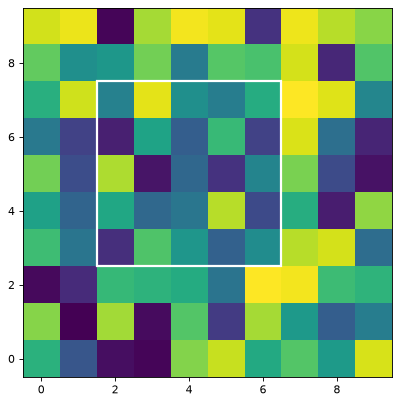# BoundingBox¶

class photutils.aperture.BoundingBox(ixmin, ixmax, iymin, iymax)[source]

Bases: object

A rectangular bounding box in integer (not float) pixel indices.

Parameters
ixmin, ixmax, iymin, iymaxint

The bounding box pixel indices. Note that the upper values (iymax and ixmax) are exclusive as for normal slices in Python. The lower values (ixmin and iymin) must not be greater than the respective upper values (ixmax and iymax).

Examples

>>> from photutils import BoundingBox

>>> # constructing a BoundingBox like this is cryptic:
>>> bbox = BoundingBox(1, 10, 2, 20)

>>> # it's better to use keyword arguments for readability:
>>> bbox = BoundingBox(ixmin=1, ixmax=10, iymin=2, iymax=20)
>>> bbox  # nice repr, useful for interactive work
BoundingBox(ixmin=1, ixmax=10, iymin=2, iymax=20)

>>> # sometimes it's useful to check if two bounding boxes are the same
>>> bbox == BoundingBox(ixmin=1, ixmax=10, iymin=2, iymax=20)
True
>>> bbox == BoundingBox(ixmin=7, ixmax=10, iymin=2, iymax=20)
False

>>> # "shape" and "slices" can be useful when working with numpy arrays
>>> bbox.shape  # numpy order: (y, x)
(18, 9)
>>> bbox.slices  # numpy order: (y, x)
(slice(2, 20, None), slice(1, 10, None))

>>> # "extent" is useful when plotting the BoundingBox with matplotlib
>>> bbox.extent  # matplotlib order: (x, y)
(0.5, 9.5, 1.5, 19.5)


Attributes Summary

 extent The extent of the mask, defined as the (xmin, xmax, ymin, ymax) bounding box from the bottom-left corner of the lower-left pixel to the upper-right corner of the upper-right pixel. shape The (ny, nx) shape of the bounding box. slices The bounding box as a tuple of slice objects.

Methods Summary

 as_artist(**kwargs) Return a matplotlib.patches.Rectangle that represents the bounding box. as_patch(**kwargs) Deprecated since version 0.7. from_float(xmin, xmax, ymin, ymax) Return the smallest bounding box that fully contains a given rectangle defined by float coordinate values. intersection(other) Return a BoundingBox representing the intersection of this BoundingBox with another BoundingBox. plot([axes, origin]) Plot the BoundingBox on a matplotlib Axes instance. Return a RectangularAperture that represents the bounding box. union(other) Return a BoundingBox representing the union of this BoundingBox with another BoundingBox.

Attributes Documentation

extent

The extent of the mask, defined as the (xmin, xmax, ymin, ymax) bounding box from the bottom-left corner of the lower-left pixel to the upper-right corner of the upper-right pixel.

The upper edges here are the actual pixel positions of the edges, i.e., they are not “exclusive” indices used for python indexing. This is useful for plotting the bounding box using Matplotlib.

shape

The (ny, nx) shape of the bounding box.

slices

The bounding box as a tuple of slice objects.

The slice tuple is in numpy axis order (i.e., (y, x)) and therefore can be used to slice numpy arrays.

Methods Documentation

as_artist(**kwargs)[source]

Return a matplotlib.patches.Rectangle that represents the bounding box.

Parameters
kwargsdict

Any keyword arguments accepted by matplotlib.patches.Patch.

Returns
resultmatplotlib.patches.Rectangle

A matplotlib rectangular patch.

Examples

import matplotlib.pyplot as plt
from photutils import BoundingBox
bbox = BoundingBox(2, 7, 3, 8)
fig = plt.figure()
rng = np.random.default_rng(0)
ax.imshow(rng.random((10, 10)), interpolation='nearest',
cmap='viridis')
lw=2.))as_patch(**kwargs)[source]

Deprecated since version 0.7: The as_patch function is deprecated and may be removed in a future version. Use as_artist instead.

Return a matplotlib.patches.Rectangle that represents the bounding box.

Parameters
kwargsdict

Any keyword arguments accepted by matplotlib.patches.Patch.

Returns
resultmatplotlib.patches.Rectangle

A matplotlib rectangular patch.

classmethod from_float(xmin, xmax, ymin, ymax)[source]

Return the smallest bounding box that fully contains a given rectangle defined by float coordinate values.

Following the pixel index convention, an integer index corresponds to the center of a pixel and the pixel edges span from (index - 0.5) to (index + 0.5). For example, the pixel edge spans of the following pixels are:

• pixel 0: from -0.5 to 0.5

• pixel 1: from 0.5 to 1.5

• pixel 2: from 1.5 to 2.5

In addition, because BoundingBox upper limits are exclusive (by definition), 1 is added to the upper pixel edges. See examples below.

Parameters
xmin, xmax, ymin, ymaxfloat

Float coordinates defining a rectangle. The lower values (xmin and ymin) must not be greater than the respective upper values (xmax and ymax).

Returns
bboxBoundingBox object

The minimal BoundingBox object fully containing the input rectangle coordinates.

Examples

>>> from photutils import BoundingBox
>>> BoundingBox.from_float(xmin=1.0, xmax=10.0, ymin=2.0, ymax=20.0)
BoundingBox(ixmin=1, ixmax=11, iymin=2, iymax=21)

>>> BoundingBox.from_float(xmin=1.4, xmax=10.4, ymin=1.6, ymax=10.6)
BoundingBox(ixmin=1, ixmax=11, iymin=2, iymax=12)

intersection(other)[source]

Return a BoundingBox representing the intersection of this BoundingBox with another BoundingBox.

Parameters
otherBoundingBox

The BoundingBox to intersect with this one.

Returns
resultBoundingBox

A BoundingBox representing the intersection of the input BoundingBox with this one.

plot(axes=None, origin=0, 0, **kwargs)[source]

Plot the BoundingBox on a matplotlib Axes instance.

Parameters
axesmatplotlib.axes.Axes or None, optional

The matplotlib axes on which to plot. If None, then the current Axes instance is used.

originarray_like, optional

The (x, y) position of the origin of the displayed image.

kwargsdict

Any keyword arguments accepted by matplotlib.patches.Patch.

to_aperture()[source]

Return a RectangularAperture that represents the bounding box.

union(other)[source]

Return a BoundingBox representing the union of this BoundingBox with another BoundingBox.

Parameters
otherBoundingBox

The BoundingBox to join with this one.

Returns
resultBoundingBox

A BoundingBox representing the union of the input BoundingBox with this one.